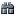### Class/Module IndexQuicksearch

The `Thread::Queue` class implements multi-producer, multi-consumer queues. It is especially useful in threaded programming when information must be exchanged safely between multiple threads. The `Thread::Queue` class implements all the required locking semantics.

The class implements FIFO type of queue. In a FIFO queue, the first tasks added are the first retrieved.

Example:

```queue = Thread::Queue.new

producer = Thread.new do
5.times do |i|
sleep rand(i) # simulate expense
queue << i
puts "#{i} produced"
end
end

consumer = Thread.new do
5.times do |i|
value = queue.pop
sleep rand(i/2) # simulate expense
puts "consumed #{value}"
end
end

consumer.join
```

### Public Class Methods

Thread::Queue.new → empty_queue click to toggle source

Creates a new queue instance, optionally using the contents of an `enumerable` for its initial state.

Example:

```q = Thread::Queue.new
q.empty?
#=> true

q = Thread::Queue.new([1, 2, 3])
q.empty?
#=> false
q.pop
#=> 1
```
```
static VALUE
rb_queue_initialize(int argc, VALUE *argv, VALUE self)
{
VALUE initial;
struct rb_queue *q = queue_ptr(self);
if ((argc = rb_scan_args(argc, argv, "01", &initial)) == 1) {
initial = rb_to_array(initial);
}
RB_OBJ_WRITE(self, &q->que, ary_buf_new());
if (argc == 1) {
rb_ary_concat(q->que, initial);
}
return self;
}
```

### Public Instance Methods

<<(p1) click to toggle source
Alias for: push
clear() click to toggle source

Removes all objects from the queue.

```
static VALUE
rb_queue_clear(VALUE self)
{
struct rb_queue *q = queue_ptr(self);

rb_ary_clear(check_array(self, q->que));
return self;
}
```
close click to toggle source

Closes the queue. A closed queue cannot be re-opened.

After the call to close completes, the following are true:

• `closed?` will return true

• `close` will be ignored.

• calling enq/push/<< will raise a `ClosedQueueError`.

• when `empty?` is false, calling deq/pop/shift will return an object from the queue as usual.

• when `empty?` is true, deq(false) will not suspend the thread and will return nil. deq(true) will raise a `ThreadError`.

`ClosedQueueError` is inherited from `StopIteration`, so that you can break loop block.

Example:

```q = Thread::Queue.new
while e = q.deq # wait for nil to break loop
# ...
end
}
q.close
```
```
static VALUE
rb_queue_close(VALUE self)
{
struct rb_queue *q = queue_ptr(self);

if (!queue_closed_p(self)) {
FL_SET(self, QUEUE_CLOSED);

wakeup_all(queue_waitq(q));
}

return self;
}
```
closed? click to toggle source

Returns `true` if the queue is closed.

```
static VALUE
rb_queue_closed_p(VALUE self)
{
return RBOOL(queue_closed_p(self));
}
```
deq(*args) click to toggle source
Alias for: pop
empty? click to toggle source

Returns `true` if the queue is empty.

```
static VALUE
rb_queue_empty_p(VALUE self)
{
return RBOOL(queue_length(self, queue_ptr(self)) == 0);
}
```
enq(p1) click to toggle source
Alias for: push
length click to toggle source
size

Returns the length of the queue.

```
static VALUE
rb_queue_length(VALUE self)
{
return LONG2NUM(queue_length(self, queue_ptr(self)));
}
```
Also aliased as: size
marshal_dump() click to toggle source
```
static VALUE undumpable(VALUE obj))
```
num_waiting() click to toggle source

Returns the number of threads waiting on the queue.

```
static VALUE
rb_queue_num_waiting(VALUE self)
{
struct rb_queue *q = queue_ptr(self);

return INT2NUM(q->num_waiting);
}
```
pop(non_block=false) click to toggle source
deq(non_block=false)
shift(non_block=false)

Retrieves data from the queue.

If the queue is empty, the calling thread is suspended until data is pushed onto the queue. If `non_block` is true, the thread isn't suspended, and `ThreadError` is raised.

```
static VALUE
rb_queue_pop(int argc, VALUE *argv, VALUE self)
{
int should_block = queue_pop_should_block(argc, argv);
return queue_do_pop(self, queue_ptr(self), should_block);
}
```
Also aliased as: deq, shift
push(object) click to toggle source
enq(object)
<<(object)

Pushes the given `object` to the queue.

```
static VALUE
rb_queue_push(VALUE self, VALUE obj)
{
return queue_do_push(self, queue_ptr(self), obj);
}
```
Also aliased as: enq, <<
shift(*args) click to toggle source
Alias for: pop
size() click to toggle source
Alias for: length